Mathematics
Easy

Question

# The slope of the line in the graph is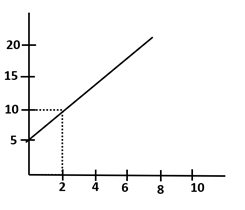## 522.510Hint:

## The correct answer is: 2.5

### In the question from the given graph we have to find the slope of the line.From the graph, we can say that the points along the line are (0,5) and (2,10).Let (0,5) and (2,10) be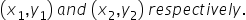Put the values of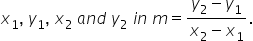So, the slope of the line joining the points (0,5) and (2,10) is 2.5.Therefore the correct option is c, i.e., 2.5.

Slope of a line shows the inclination of a line. We can find the slope of a line using the points of the line using the formula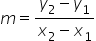, where m is the slope of the line,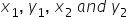are the coordinates of the two points.#### With Turito Foundation.#### Get an Expert Advice From Turito.